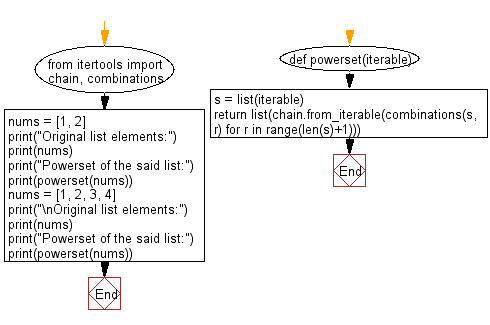﻿ Python: Find the powerset of a given iterable - w3resource# Python: Find the powerset of a given iterable

## Python List: Exercise - 256 with Solution

Write a Python program to get the powerset of a given iterable.

Note: A Power Set is a set of all the subsets of a set.

• Use list() to convert the given value to a list.
• Use range() and itertools.combinations() to create a generator that returns all subsets.
• Use itertools.chain.from_iterable() and list() to consume the generator and return a list.

Sample Solution:

Python Code:

``````from itertools import chain, combinations
def powerset(iterable):
s = list(iterable)
return list(chain.from_iterable(combinations(s, r) for r in range(len(s)+1)))
nums = [1, 2]
print("Original list elements:")
print(nums)
print("Powerset of the said list:")
print(powerset(nums))
nums = [1, 2, 3, 4]
print("\nOriginal list elements:")
print(nums)
print("Powerset of the said list:")
print(powerset(nums))
```
```

Sample Output:

```Original list elements:
[1, 2]
Powerset of the said list:
[(), (1,), (2,), (1, 2)]

Original list elements:
[1, 2, 3, 4]
Powerset of the said list:
[(), (1,), (2,), (3,), (4,), (1, 2), (1, 3), (1, 4), (2, 3), (2, 4), (3, 4), (1, 2, 3), (1, 2, 4), (1, 3, 4), (2, 3, 4), (1, 2, 3, 4)]
```

Flowchart:## Visualize Python code execution:

The following tool visualize what the computer is doing step-by-step as it executes the said program:

Python Code Editor:

Have another way to solve this solution? Contribute your code (and comments) through Disqus.

What is the difficulty level of this exercise?

Test your Python skills with w3resource's quiz

﻿

## Python: Tips of the Day

Floor Division:

When we speak of division we normally mean (/) float division operator, this will give a precise result in float format with decimals.

For a rounded integer result there is (//) floor division operator in Python. Floor division will only give integer results that are round numbers.

```print(1000 // 300)
print(1000 / 300)```

Output:

```3
3.3333333333333335```